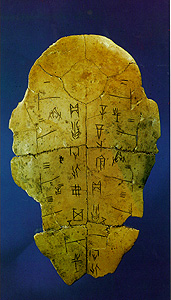### Chinese Mathematics

Chinese mathematics, was defined by Chinese in ancient times as the "art of calculation" (suan chu). This art was both a practical and spiritual one, and covered a wide range of subjects from religion and astronomy to water control and administration.

The first true evidence of mathematical activity in China can be found in numeration symbols on tortoise shells and flat cattle bones (commonly called oracle bones, dated from the Shang dynasty. (14th century B.C.)These numerical inscriptions contain both tally and code symbols which are based on a decimal system, and they employed a positional value system. This proves that the Chinese were one of the first civilization to understand and efficiently use a decimal numeration system. Early Chinese mathematics had a great influence on other later civilizations, in India, Japan, Korea and other counties.

The numeration system used in the modern world had its origins 34 centuries ago in Shang China. However, mathematics in China began much earlier in the development of the Chinese calendar, flood-control measures, administration, and so on.

The need to control the flood-prone rivers of China, such as the Yangtze and Yellow Rivers, was an important factor in the development of mathematics in ancient China. The problem of providing a safe environment in a water-dependent society were solved using science and mathematics, including the construction of canals, dams, etc.

According to legend, a mythological Emperor Yu received a divine gift from a Lo river tortoise. The gift was in the form of diagrams called Lo shu, which was believed to contain the principles of Chinese mathematics. One diagram, the magic square, was thought to possess magical qualities, and led to the development of the dualistic theory of Yin and Yang Yin represents even numbers (2, 4, 6, 8, 10...) and Yang represents odd numbers. (1, 3, 5, 7, 9, 11...)

The Chinese also discovered the concept of Zero and invented a wide variety of mechanical aids like counting boards, and wrote numerous mathematics texts to aid them in mathematical calculation.

The oldest existing Chinese texts containing formal mathematical theories were produced during the Han period. The Arithmetic Classic of the Gnomon and the Circular Paths of Heaven (Chou Pei Suan Ching) is dated before the 3rd century B.C and contains various modern mathematical principles such as working with fractions using a common denominator, and proofs of many geometrical theories. The text contains an accurate process of division for finding out the square root of numbers.

In fact, the Chou Pei presents the oldest known proof of the right-angle triangle theory in the hsuan-thu diagram. This theory, commonly known as the "Pythagorean theorem," shows that the sum of the squares of the legs of a right triangle is equal to the squares of the hypotenuse or (A X 2) + (B X 2) = (C X 2).

CHINA INDEXSearch crystalinks.com Search web